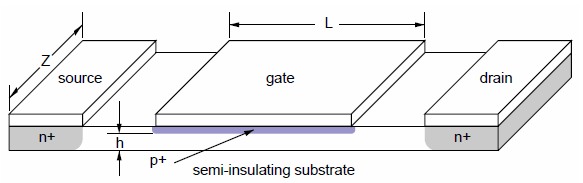PHT.301 Physics of Semiconductor Devices

Consider and $n$-channel JFET.A JFET consists of a semiconducting channel contacted by two ohmic contacts. The gate contacts a heavily doped $p$-region above the channel. The current in the channel flows between the $pn$-junction and a semi-insulating substrate. When the $pn$-junction is reverse biased, the depletion width expands and the channel becomes narrower. The thickness of the conducting channel is $h-x_n(y)$ where $h$ is the distance from the metalurgical junction (where the doping changes from $p$ to $n$) to the semi-insulating substrate and $x_n(y)$ is the depletion width that depends on the position $y$ along the channel. In the figure below, the gray region of the channel is depleted.A constant current $I_D$ flows from the drain to the source. The voltage drop across a short section of the channel is $dV = I_DR$ where $R$ is the resistance of this short section. Ohm's law states,

$\begin{equation} R = \frac{\rho dy}{(h-x_n(y))Z}. \end{equation}$

For an $n$-doped extrinsic semiconductor, the resistivity is $\rho=1/(e\mu_nN_D)$ where $-e$ is the charge of an electron, $\mu_n$ is the electron mobility, and $N_D$ is the donor concentration. This can be combined to yield,

$\begin{equation} I_Ddy = e\mu_nN_DZ(h-x_n(y))dV \end{equation}$

The formula for the depletion width of a one-sided $pn$-junction is,

$\begin{equation} x_n = \sqrt{\frac{2\epsilon_r\epsilon_0(V_{bi}-V)}{eN_D}}, \end{equation}$

where $V_{bi}$ is the built-in voltage and $V$ is the forward bias voltage across the junction. In the case of a JFET, a positive voltage on the gate forward biases the junction and a positive voltage on the drain reverse biases the junction so the depletion with is,

$\begin{equation} x_n(y) = \sqrt{\frac{2\epsilon_r\epsilon_0(V_{bi}+V(y)-V_G)}{eN_D}}, \end{equation}$

where $V(y)$ is the potential along the channel. Differentiating this expression we find,

$\begin{equation} \frac{dx_n(y)}{dV} = \frac{1}{2\sqrt{\frac{2\epsilon_r\epsilon_0(V_{bi}+V(y)-V_G)}{eN_D}}}\frac{2\epsilon_r\epsilon_0}{eN_D}=\frac{\epsilon_r\epsilon_0}{eN_Dx_n(y)}. \end{equation}$

Thus, $\begin{equation} dV =\frac{eN_Dx_n}{\epsilon_r\epsilon_0}dx_n. \end{equation}$

Substituting this into Eq. 2 yields a first order differential equation that can be solved for the depletion width as a function of position along the channel.

$\begin{equation} I_Ddy = \frac{e^2\mu_nN_D^2Z}{\epsilon_r\epsilon_0}(h-x_n)x_ndx_n. \end{equation}$

Integrating from source to drain,

$\begin{equation} I_D\int\limits_0^L dy = \frac{e^2\mu_nN_D^2Z}{\epsilon_r\epsilon_0}\int\limits_{x_L}^{x_R}(h-x_n)x_ndx_n. \end{equation}$

The depletion width at the source is $x_L$, the depletion width at the drain is $x_R$.

$\begin{equation} \frac{I_D\epsilon_r\epsilon_0L}{e^2\mu_nN_D^2Z}= \frac{hx_R^2}{2} -\frac{x_R^3}{3}-\frac{hx_L^2}{2} +\frac{x_L^3}{3}. \end{equation}$

Using the expresions for the depletion widths at the source and the drain and the definition of the pinch-off voltage $V_p$,

$\begin{equation} x_L = \sqrt{\frac{2\epsilon_r\epsilon_0(V_{bi}-V_G)}{eN_D}},\qquad x_R = \sqrt{\frac{2\epsilon_r\epsilon_0(V_{bi}+V_D-V_G)}{eN_D}},\qquad h = \sqrt{\frac{2\epsilon_r\epsilon_0V_p}{eN_D}}, \end{equation}$

the drain current in the linear regime can be expressed in terms of the gate voltage and drain voltage,

$\begin{equation} \boxed{I_D=I_p\left[\frac{V_D}{V_p}-\frac{2}{3}\left(\frac{V_{bi}+V_D-V_G}{V_p}\right)^{3/2}+\frac{2}{3}\left(\frac{V_{bi}-V_G}{V_p}\right)^{3/2}\right].} \end{equation}$

Here,

\begin{align} I_p =\frac{\mu_n {N_D}^2 Z e^2 h^3}{2L\epsilon_r\epsilon_0}, \qquad V_p =\frac{e {N_D} h^2}{2 \epsilon_r\epsilon_0}, \qquad eV_{bi} =k_B T \ln \left(\frac{N_A N_D}{{n_i}^2}\right), \qquad n_i=\sqrt{N_cN_v\left(\frac{T}{300}\right)^{3}}\exp\left(\frac{-E_g}{2k_BT}\right). \end{align}

Eq. 11 for the drain is valid in the linear regime before the channel is pinched-off. After pinch-off the JFET is in the saturation regime and the current is,

$\begin{equation} I_{D,sat}=I_p\left[\frac{1}{3}-\frac{V_{bi}-V_G}{V_p}+\frac{2}{3}\left(\frac{V_{bi}-V_G}{V_p}\right)^{3/2}\right]. \end{equation}$

This derivation assumed that the $pn$ junction is reverse biased. For a n-channel JFET, $V_G$ < 0 and $V_D$ > 0 in this regime. The gray area of the plot below shows the part of the channel that is depleted.

 $h - x_n$ [μm] $y$ [μm]
 $N_c=$ cm-3 @ 300 K $N_v=$ cm-3 @ 300 K $E_g=$ eV $N_D=$ cm-3 $N_A=$ cm-3 $\mu_n=$ cm2/Vs $h=$ μm $L=$ μm $Z=$ μm $\epsilon_r=$ $T=$ K $V_{D}$ = V $V_g$ = V

The electric field along the channel is,

\begin{equation} E_y = -\frac{dV}{dy} =- \frac{I_D}{e\mu_nN_DZ(h-x_n(y))}. \end{equation}

In the gradual channel approximation, the electric field diverges at pinch-off where $h=x_n(y)$. However, in the depleted section of the channel the electric field should be calculated with Gauss's law $\frac{dE_x}{dx}+\frac{dE_y}{dy}=\frac{\rho}{\epsilon_r\epsilon_0}$. The derivative of the field cannot exceed $\frac{eN_D}{\epsilon_r\epsilon_0}$, in the depleted regions so the electric field remains finite near the drain.

 $E_y$ [kV/m] $y$ [μm]

$E_g=$  eV;  $n_i=$  cm-3;  $V_{bi}=$  V;  $I_p=$  mA;  $V_p=$  V $I_d=$  mA $x_L=$  μm $x_R=$  μm,  $\frac{eN_D}{\epsilon_r\epsilon_0}=$  kV/m².